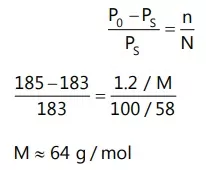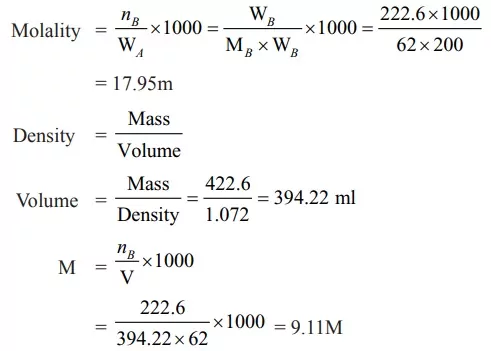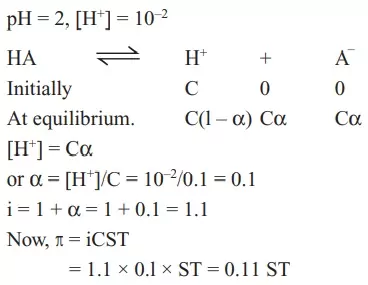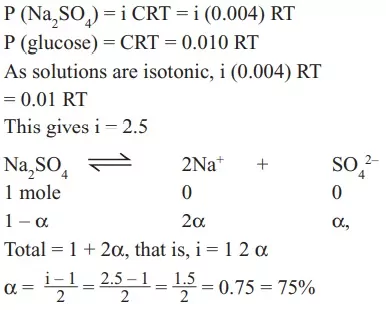# Solutions Chemistry Class 12 MCQSolutions Chemistry Class 12 MCQ

Que 1. If sodium sulphate is considered to be completely dissociated into cations and anions in an aqueous solution, the change in freezing point of water(∆Tf ), when 0.01 mol of sodium sulphate is dissolved in 1 kg of water, is (Kf = 1.86 K kg mol-1)
(A) 0.0372 K                                                              (B) 0.0558 K
(C) 0.0744 K                                                              (D) 0.0186 K
Ans 1. (B)
Reason: Van’t Hoff factor (i) for Na2SO4 = 3
ΔTf = i Kf m = 3 × 1.86 × 0.01 = 0.0558

Que 2. The vapour pressure of acetone at 20°C is 185 torr. When 1.2 g of a non-volatile substance was dissolved in 100 g of acetone a 20°C, its vapour pressure was 183 torr. The molar mass (g mol-1) of the substance is:
(A) 32                                                                         (B) 64
(C) 128                                                                       (D) 488
Ans 2. (B)
Reason: We knowQue 3. Acetone and carbon disulphide form binary liquid solution showing positive deviation from Raoult’s law. The normal boiling point (Tb) of pure acetone is less than that of pure CS2. Pick out the incorrect statement among the following
(A) Boiling temperature of the mixture is always less than the boiling temperature of acetone
(B) Boiling temperature of Azetropic mixture is always less than the boiling temperature of acetone
(C) When a small amount of CS2 (less volatile component) is added to an excess of acetone boiling point of the resulting mixture increases
(D) A mixture of CS2 and CH3 COCH3 can be completely separated by simple fractional distillation
Ans 3. (D)
Reason: A mixture of CS2 and CH3 COCH3 can be completely separated by simple fractional distillation.

Solutions Chemistry Class 12 MCQ

Que 4. An antifreeze solution is prepared from 222.6 g of ethylene glycol C2H4(OH)2 and 200 g of water. Calculate the molality of the solution. If the density of this solution be 1.072 g mL-1, what will be the molarity of the solution?
(a) 7.20 M                               (b) 12.03 M
(c) 9.11 M                               (d) 6 M
Ans 4. (c)
Reason: MB of C2H4(OH)2 = 62 g/molQue 5. Which of the following solutions have the highest boiling point and why?
(a) 1M glucose                (b) 1M KCl                (c) 1M aluminium nitrate
Ans 5. (c)
Reason: 1 m glucose
Value of i = 1  (for non-electrolytes)
So, molal concentration will be = 1 × 1 = 1m
1 m KCl
Value of i = 2
So, molal concentration will be = 1 × 2 = 2m
1 m Al(NO3)3
Value of i = 4
So, molal concentration will be = 1 × 4 = 4m

Que 6. Which one of the following statements is false?
(a) Two sucrose solutions of the same molality prepared in different solvents will have the same freezing point depression
(b) The osmotic pressure (π) of a solution is given by the equation π = MRT, where M is the molarity of the solution
(c) Raoult’s law states that the vapour pressure of a component over a solution is proportional to its mole fraction
(d) The correct order of osmotic pressure for 0.01 M aqueous solution of each compound is BaCl2 > KCl > CH3COOH > Sucrose
Ans 6. (a)
Reason: Two sucrose solutions of the same molality prepared in different solvents will have the same freezing point depression.

Solutions Chemistry Class 12 MCQ

Que 7. A 5.25% solution of a substance is isotonic with a 1.5% solution of urea (molar mass = 60 g mol–1) in the same solvent. If the densities of both the solutions are assumed to be equal to 1.0 g cm–3, the molar mass of the substance will be:
(a) 115.0 g mol–1                                            (b) 105.0 g mol–1
(c) 210.0 g mol–1                                            (d) 90.0 g mol–1
Ans 7. (c)
Reason: Solutions with the same osmotic pressure are called isotonic. Let the molar mass of the substance be M
π1 = π2 C1 RT = C2 RT
So, C1 = C2 As density of the solutions are the same

Que 8. When mercuric iodide is added to the aqueous solution of potassium iodide, the:
(a) Freezing point is raised
(b) Freezing point does not change
(c) Freezing point is lowered
(d) Boiling point does not change
Ans 8. (a)
Reason: 2KI + HgI2 → K2[HgI4] ↔ 2K+ + [HgI4]–2 there is a decrease in the number of ions present in the solution so ΔTf decrease or freezing point is raised.

Que 9. pH of 0.1 M monobasic acid solution is found to be 2. Thus its osmotic pressure at T. Kelvin is:
(a) 11.11 ST                                       (b) 0.11 ST
(c) 0.011 ST                                        (d) 1.11 S
Ans 9. (b)
Reason:Que 10. A 0.004 M solution of Na2SO4 is isotonic with a 0.010 M solution of glucose at same temperature. The apparent degree of dissociation of Na2SO4 is:
(a) 25%                                               (b) 50%
(c) 75%                                               (d) 85%
Ans 10. (c)
Reason:Solutions Chemistry Class 12 MCQ